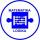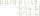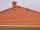# AP - basics

Determine first member and differentiate of the the following sequence:

a3-a5=24
a4-2a5=61

Result

a =  -1
d =  -12

#### Solution:

a+2d -(a+4d) = 24
a+3d - 2(a+4d) = 61

2d = -24
a+5d = -61

a = -1
d = -12

Calculated by our linear equations calculator.

Leave us a comment of example and its solution (i.e. if it is still somewhat unclear...):

Showing 0 comments:Be the first to comment!#### To solve this example are needed these knowledge from mathematics:

Do you have a system of equations and looking for calculator system of linear equations?

## Next similar examples:

1. Third memberDetermine the third member of the AP if a4=93, d=7.5.
2. AS sequenceIn an arithmetic sequence is given the difference d = -3 and a71 = 455. a) Determine the value of a62 b) Determine the sum of 71 members.
3. Sequence 3Write the first 5 members of an arithmetic sequence: a4=-35, a11=-105.
4. AS - sequenceWhat are the first ten members of the sequence if a11=22, d=2.
5. Sequence 2Write the first 5 members of an arithmetic sequence a11=-14, d=-1
6. SequenceWrite the first 7 members of an arithmetic sequence: a1=-3, d=6.
7. SeatsSeats in the sport hall are organized so that each subsequent row has five more seats. First has 10 seats. How many seats are: a) in the eighth row b) in the eighteenth row
8. Gasoline canisters35 liters of gasoline is to be divided into 4 canisters so that in the third canister will have 5 liters less than in the first canister, the fourth canister 10 liters more than the third canister and the second canister half of that in the first canist
9. Three workshopsThere are 2743 people working in three workshops. In the second workshop works 140 people more than in the first and in third works 4.2 times more than the second one. How many people work in each workshop?
10. Inverse matrixFind out inverse by Gauss elimination or by reduction method. A=[2/3. 1 -3. 1/3]
11. SequenceBetween numbers 1 and 53 insert n members of the arithmetic sequence that its sum is 702.
12. Theorem proveWe want to prove the sentense: If the natural number n is divisible by six, then n is divisible by three. From what assumption we started?
13. Linsys2Solve two equations with two unknowns: 400x+120y=147.2 350x+200y=144
14. Three unknownsSolve the system of linear equations with three unknowns: A + B + C = 14 B - A - C = 4 2A - B + C = 0
15. LegsCancer has 5 pairs of legs. The insect has 6 legs. 60 animals have a total of 500 legs. How much more are cancers than insects?
16. RoofTiles are stacked in rows on the trapezoidal shaped roof. At the ridge is 15 tiles and each subsequent row has one more tile than in the previous row. How many tiled is covered roof if lowermost row has 37 tiles?
17. Elimination methodSolve system of linear equations by elimination method: 5/2x + 3/5y= 4/15 1/2x + 2/5y= 2/15